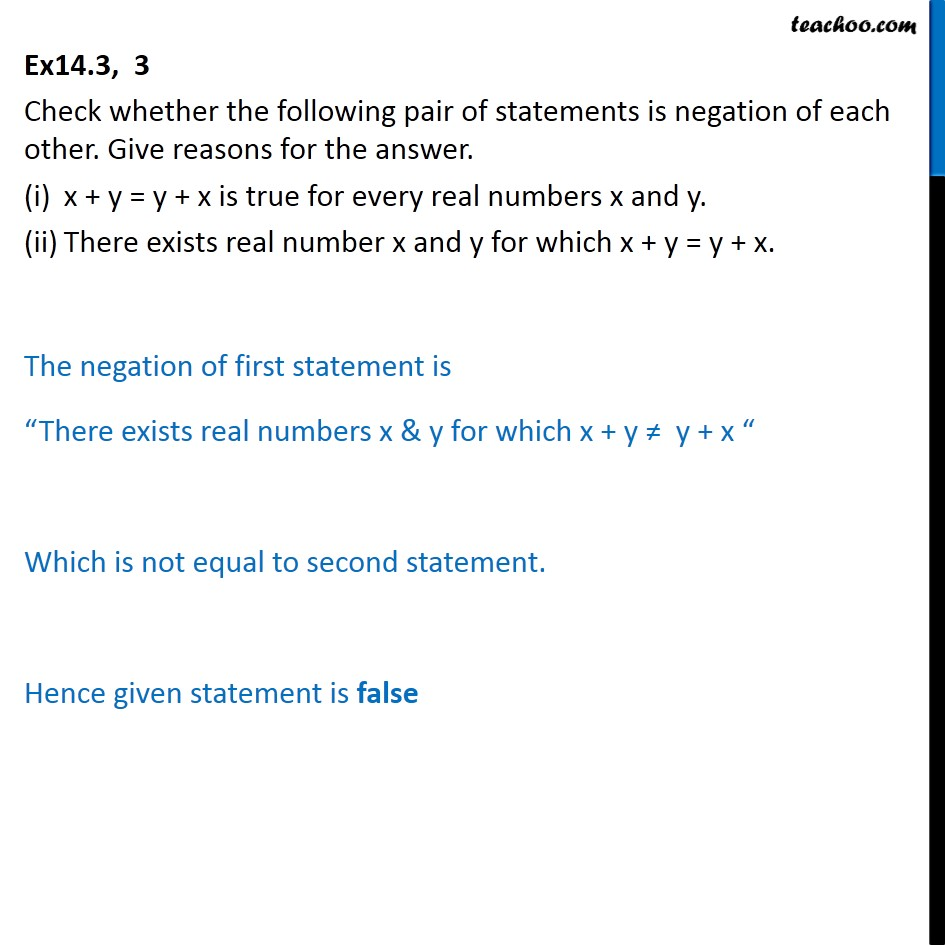Compound Statements

Mathematical Reasoning
Serial order wiseLearn in your speed, with individual attention - Teachoo Maths 1-on-1 Class

### Transcript

Question 3 Check whether the following pair of statements is negation of each other. Give reasons for the answer. x + y = y + x is true for every real numbers x and y. There exists real number x and y for which x + y = y + x. The negation of first statement is There exists real numbers x & y for which x + y y + x Which is not equal to second statement. Hence given statement is false